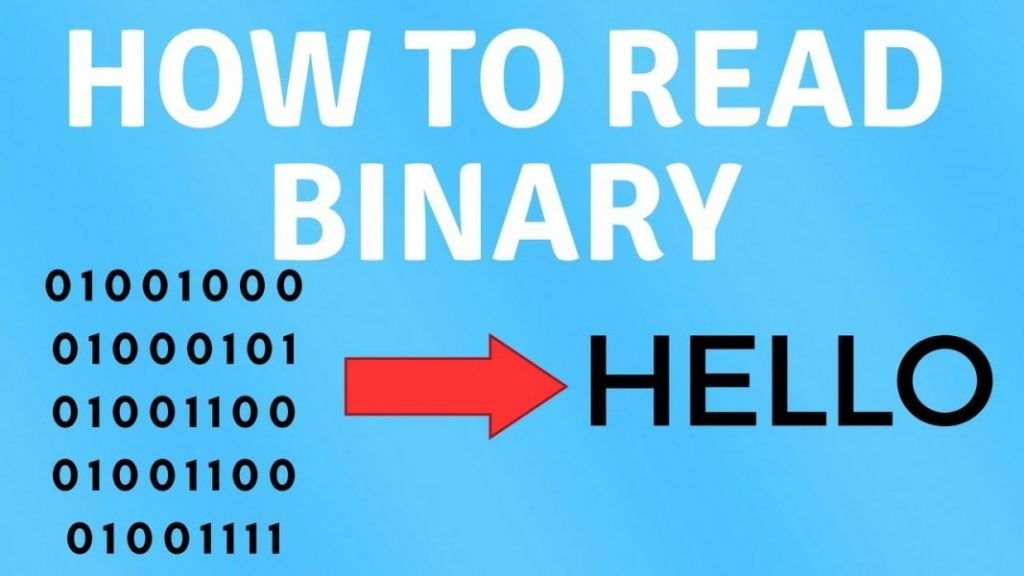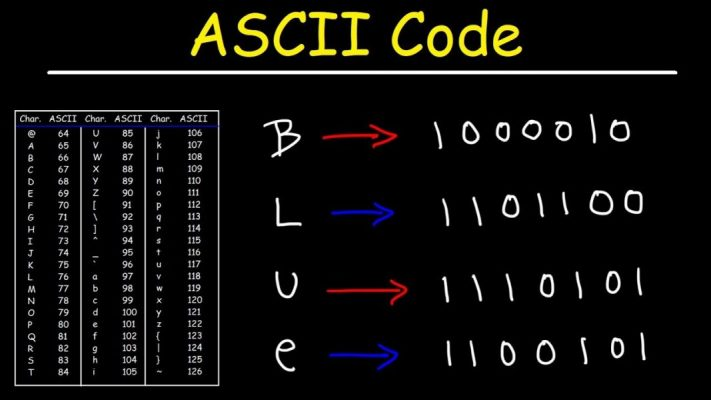# Binary Numbers and Ways for Accurate ConversionsHave you ever thought about the use of binary numbers? If the answer to this question is no, then you need to know that it served as the foundation for the technological advancements we are witnessing today. Binary numbers were used to form the machine language that controls computers, smart devices, and digital wiring. Computers and machines are not capable of understanding or interpreting our language just like other humans do. The only way for machines to understand instructions and process, interpret and retain data is the conversion of data into the machine language, which is based on binary numbers.

The binary number system is quite simple if we compare it to the decimal number system. This number system only contains two characters or digits that are 0 and 1. The base of this number system is 2, and it was introduced and developed by Gottfried Wilhelm Leibniz. The simplicity of this number system was the main reason behind its use as the foundation of machine language. Simply put, the character ‘0’ in machine language represents the ‘off’ status, while 1 reflects the ‘on’ status. However, if we humans want to understand the machine language, then we need to convert data written in binary to text. Read on to know more details about binary to text conversions.

## Why do We Need to Convert Binary to Text and Other Forms?

Before we move on to discuss various methods of converting binary to text, it is essential to understand how the need for such a conversion arises. We have outlined some scenarios where the binary translation is needed. Further details are given below:

### Machine Language is Difficult to Understand

As mentioned earlier, the machine language consists of batches of binary code, including various patterns of 0 and 1. You can’t understand this language easily. If you have to understand something written in the binary code, you may have to spend several hours and end up interpreting just one line. Understanding binary language in the way it is used by machines and computers is a tricky task, and most of us are not capable of that.

### Simple Arithmetic Calculations Might Take Hours

As binary language happens to be the native language of the digital ecosystem, the data is fed to computers and smart devices after getting converted into binary code. Whether you are storing simple text, images, or even videos on the computer, the files first get converted into an array of binary numbers and then get saved on the computer. When you see the output in the form of pictures, text, or arithmetic calculation, binary code is working at the back end. However, humans can’t handle big arithmetic calculations involving bigger decimal numbers when the code is written in binary form. Hence, if you want to make bigger calculations, you need to convert binary numbers into decimal numbers and then perform calculations.

## Accurate Ways of Converting Binary to Text

As reflected in the previous discussion, humans can’t handle binary code as easily and effectively as computers are capable of doing so. Hence, the best way to understand machine language or perform calculations based on the binary code is by converting it to the required form, which can be text or any other number system. Here are some accurate ways to convert binary to text and other forms:

### Manual Conversion of Binary to ASCII TextIt should be noted that binary code is not a readily convertible code to text like other codes. It requires another code like ASCII (American Standard Code for Information Interchange) before its conversion to text. How is this possible? The simple answer is the ASCII code is based on the numerical representation of different characters in decimal values. These decimal values of different characters are easily understandable by computers and smart devices. For instance, the character ‘e’ comes with numerical representation 101 in the decimal number system, according to ASCII. If we convert 101 into the binary number system, it will be 01100101. So, if you are planning to interpret a binary code, you need to convert 01100101 into a decimal number by multiplying each bit with the respective power of 2.

You can do it like this 0 x 2^7 + 1 x 2^6 + 1 x 2^5 + 0 x 2^4 + 0 x 2^3 + 1 x 2^2 + 0 x 2^1 + 1 x 2^0. Simplifying this expression will make it 1 x 64 + 1 x 32 + 1 x 4 + 1 x 1. Solving this simplified version will make it 64 + 32 + 4 + 1, which equals 101 that is ASCII representation of the character ‘e’. This is how you can interpret the binary code. Similarly, for arithmetic calculations you can convert binary to decimal and hexadecimal numbers.

### Using a Binary to Text Converter

Another relatively easy method of converting a code written in binary to text is using a binary to text converter. You can simply search for a reliable binary translator and input the binary code there; it will give you the output in text in a few minutes without any error. You don’t need to install such a tool, all you need is an internet connection, and you can easily convert binary to text.

##### End Words!

The digital ecosystem is based on machine language or binary code consisting of two characters or bits, ‘0’ and ‘1’. Although this binary code is quite simple for computers and other machines, it is not understandable by humans. If you want to understand the binary code, you need to use some accurate ways of translating binary to text form. We have discussed some effective methods for this purpose in this writing as well. We hope this information will be helpful for you!